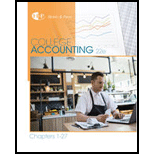# ANALY SIS OF LEVERAGE Based on the financial statement data in Exercise 24-1A, compute the following leverage measures for 20-2 (round all calculations to two decimal places): (a) Debt-to-equity ratio (b) Times interest earned ratio (Bond interest is $24,200.) (c) Assets-to-equity ratioBuyFindarrow_forward ### College Accounting, Chapters 1-27 23rd Edition HEINTZ + 1 other Publisher: Cengage Learning, ISBN: 9781337794756 #### Solutions Chapter SectionBuyFindarrow_forward ### College Accounting, Chapters 1-27 23rd Edition HEINTZ + 1 other Publisher: Cengage Learning, ISBN: 9781337794756 Chapter 24, Problem 4SEA Textbook Problem 27 views ## ANALY SIS OF LEVERAGE Based on the financial statement data in Exercise 24-1A, compute the following leverage measures for 20-2 (round all calculations to two decimal places):(a) Debt-to-equity ratio(b) Times interest earned ratio (Bond interest is$24,200.)(c) Assets-to-equity ratio

To determine

(a)

Compute debt equity ratio during the period 20-2.

### Explanation of Solution

Leverage ratio:

It overlooks the mixture of debt and equity which is required for analyzing the ability of the Company to meet its debt obligations as they become due.

Calculate debt-to equity ratio during the period 20-2.

Debt-equity ratio =Total liabilitesTotal stockholders' equity

(b)

To determine

Compute times interest earned ratio during the period 20-2.

(c)

To determine

Compute Assets to equity ratio during the period 20-2.

### Still sussing out bartleby?

Check out a sample textbook solution.

See a sample solution

#### The Solution to Your Study Problems

Bartleby provides explanations to thousands of textbook problems written by our experts, many with advanced degrees!

Get Started

Find more solutions based on key concepts
What is COSO?

Accounting Information Systems

What are the four phases in the typical business cycle?

Foundations of Business (MindTap Course List)

What is a biometric time clock?

Accounting Information Systems

EXPECTED INTEREST RATE The real risk-free rate is 3%. Inflation is expected to be 3% this year, 4% next year, a...

Fundamentals of Financial Management, Concise Edition (with Thomson ONE - Business School Edition, 1 term (6 months) Printed Access Card) (MindTap Course List)